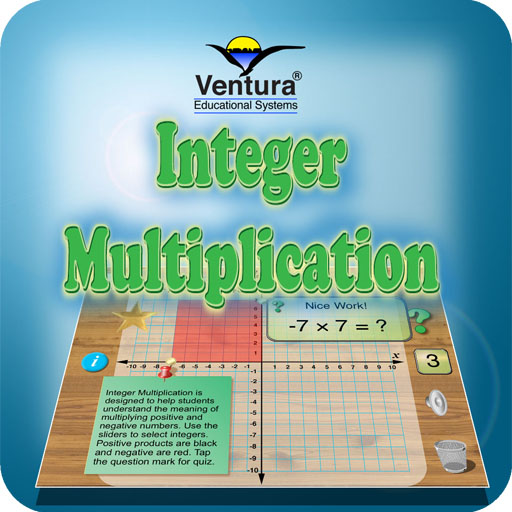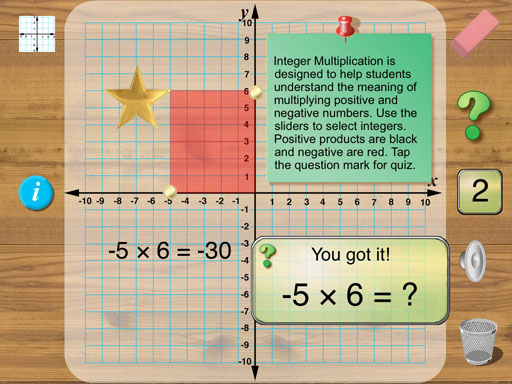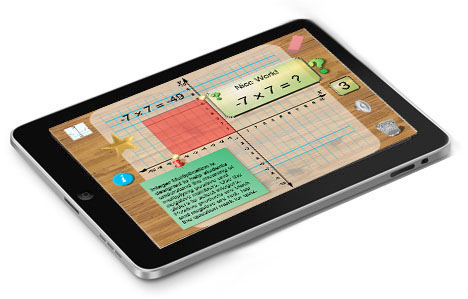A Great Way to Visualize a Difficult Math Concept!Interactive Excitement and a Great Learning Experience
 Integer Multiplication is designed to help students understand the meaning of multiplying positive and negative numbers. The set of integers is closed for multiplication and therefore all products for integers from -10 to +10 can be represented on a -10 x 10 grid. Use the gold sliders on the x and y axes to set the two numbers to be multiplied. Positive products are shown with a black rectangle and negative products are shown in red. Tap the question mark to begin a quiz. The app automatically tracks the number of correct answers. Students are motivated by the sound effects and visually appealing display.Special features make using Integer Multiplication fun: 1. Slide markers to show multiplication. 2. Random problems are automatically generated. 3. Sound effects. 4. Available for iPad iOS 7 and 8.\$1.99